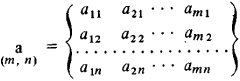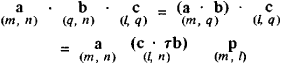# Krakowian

The following article is from The Great Soviet Encyclopedia (1979). It might be outdated or ideologically biased.

## Krakowian

a rectangular array of (m X n) elements (numbers) arranged in m columns and n rows:The Krakówian, introduced in the 1920’s by the Polish astronomer T. Banachiewicz (1882–1954), differs from ordinary matrices used in linear algebra by a simpler multiplication rule. We multiply the columns of cofactors having the same number of rows:Here rb is the transposed krakowian of b. Furthermore, operations such as division and extraction of a square root, which are lacking in matrix algebra, can be performed on Krakowians. Krakowians have found application in geodetic calculations.

### REFERENCES

Modrinskii, N. I. Primenenie krakovianov v geodezicheskikh vychisleniiakh. Moscow, 1959.
Zlatanov, G. Krakovianovo smiatane. Sofia, 1968.
Banachiewicz, T. Rachunek krakowianowy z zastosowaniami. Warsaw, 1959.

N. I. MODRINSKII